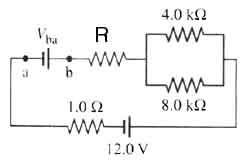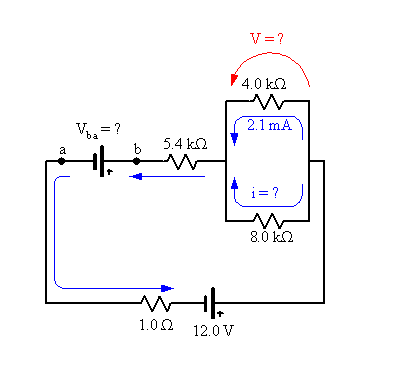# Terminal Voltage Problem

• Angie K.

## Homework StatementThe current through the 4.0 kΩ resistor in the figure above is 2.1 mA. The resistor R is 5.4 kΩ. What is the terminal voltage Vba of the "unknown" battery? (Note there are two possible answers: one positive and one negative. Give the negative voltage first.)

## Homework Equations

Vab=E-Ir
I=E/(R+r)
Kirchhoff's Laws (junction and loop rule)

The attempt at a solution

I attempted to use the equation I=E/(R+r) (converting kiloohms to ohms) and using 12V for E, 88000.9 Ohms for R and 5400 Ohms for r. Is that right, or am not supposed to use the 5400 Ohms for the little r? I'm not really sure what to do from here. I know the direction of the current matters and I chose to calculate it where the current flows from the positive end of the battery. But going from there, I'm totally lost.